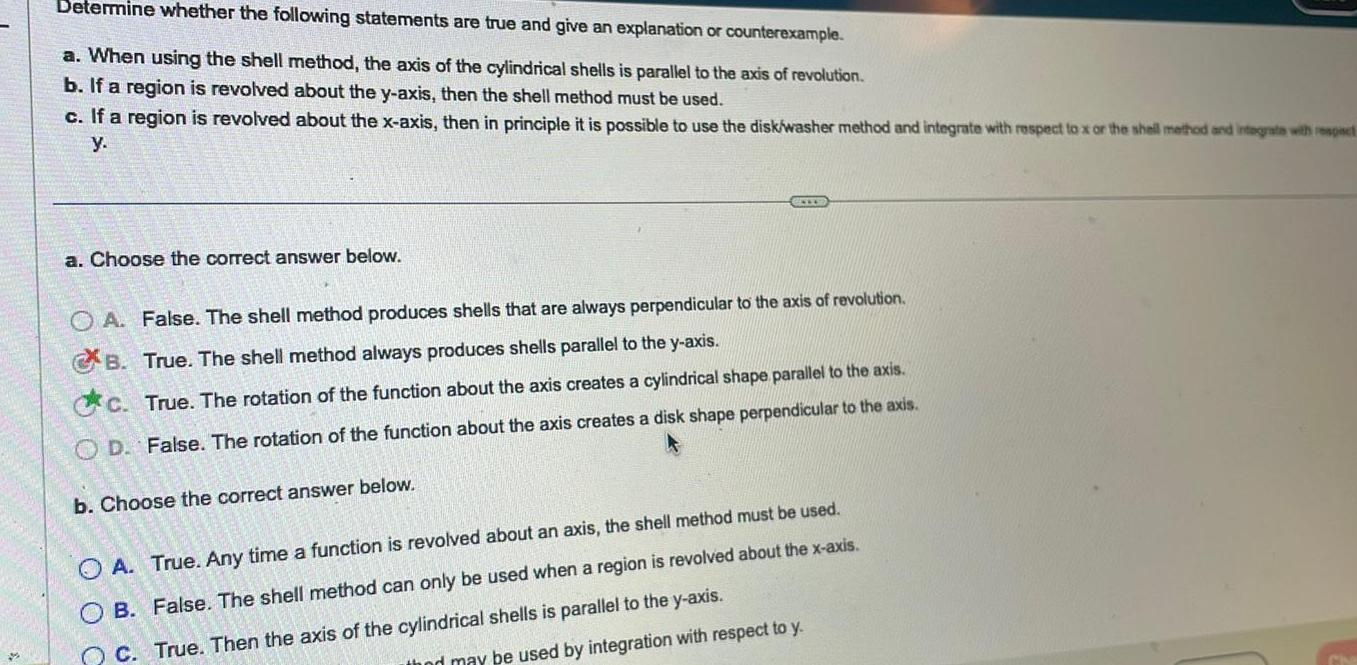Question:

# 4 Determine whether the following statements are true and

Last updated: 11/19/20234 Determine whether the following statements are true and give an explanation or counterexample a When using the shell method the axis of the cylindrical shells is parallel to the axis of revolution b If a region is revolved about the y axis then the shell method must be used c If a region is revolved about the x axis then in principle it is possible to use the disk washer method and integrate with respect to x or the shell method and integrate with respect y a Choose the correct answer below OA False The shell method produces shells that are always perpendicular to the axis of revolution True The shell method always produces shells parallel to the y axis True The rotation of the function about the axis creates a cylindrical shape parallel to the axis OD False The rotation of the function about the axis creates a disk shape perpendicular to the axis B c b Choose the correct answer below OA True Any time a function is revolved about an axis the shell method must be used OB False The shell method can only be used when a region is revolved about the x axis C True Then the axis of the cylindrical shells is parallel to the y axis thod may be used by integration with respect to y